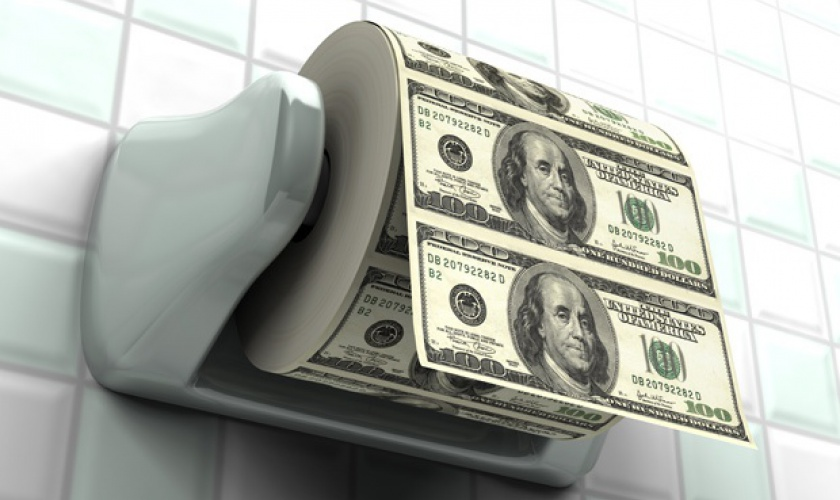# Money Handling

Previous Article Use the links to navigate to next/previous articles Next ArticleGeneral rules

1. We need to decide the amount of money which we want to have as our betting money and then spread it evenly between 3-4 trustful bookmakers, so that for every game we choose to bet something we can always choose the highest odd. It is always good to also have an account with companies like Betfair.
2. We always try to go for single bets, or the very most on bets involving 2 teams. Never more than this.
3.  We set some targets; for example to win 3 or 4 times the amount of money we have for betting. If we reach that amount then we withdraw the winnings and we are left with the original amount of money set for betting in our betting account

Equal bets

Let's suppose that we have 1000 units available for betting and that we use the same amount of money for each of our bets. Most players use 2.5% of the original amount (in this case 25 units) for each of their bets. If we win 250 units and the original amount goes up to 1250 units, we should still stick to the 2.5% rule for each bet, in this case 32.5 units; this way, when we are on the positive side of things our winnings increase by a proportionate amount whereas if we are on the negative side of things we need to keep our betting amount stable otherwise it would be harder to come to terms with the original amount available for betting.

Kelly Criterion

This is a way to increase the winnings as much as possible, on a long-term basis. More specifically, relating it to football betting, Kelly's Criterion is telling us how much money we should use for bets for which we believe the odds are higher than the real situation surrounding the game (look for the value bet explanation). Kelly's Criterion goes as follows:

A = W.C. (odd+1)-1/odd where

A: the percentage of money, from the original sum, that we want to use for a particular bet

W.C.: the chances we personally give to the bet we choose to go in favour of Odd: the chances that the bookmaker gives for any bet

Example:

Martingale or money multiplication: based on this, we choose to bet particular amount (A) of money on 1 or 2 games where the odd for the single or the multiple bet is 2.00. If we win the bet, then we use the same amount of money for our next bet, but if we lose the bet then the next time we bet we use double of the amount of money we used for our first bet. We keep repeating the process until we end up winning a bet so that when we win we end up with exactly the same amount we had before getting involved in the betting process; have a look on the table that follows:

Betting amount Winnings Profit

A 2A 2A-A=A

2A 4A 4A-(2+1)A=A

4A 8A 8A-(4+2+1)=A

8A 16A 16A-(8+4+2+1)=A

16A 32A 32A-(16+8+4+2+1)=A

32A 64A 64A-(32+16+8+4+2+1)=A